# Proof of Existence of Tensor Product ... Further Question ..

Gold Member
I am reading Bruce N. Coopersteins book: Advanced Linear Algebra (Second Edition) ... ...

I am focused on Section 10.1 Introduction to Tensor Products ... ...

I need help with another aspect of the proof of Theorem 10.1 regarding the existence of a tensor product ... ...

The relevant part of Theorem 10.1 reads as follows: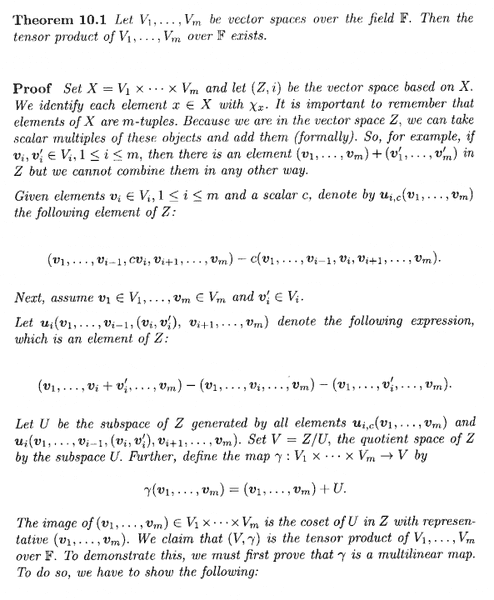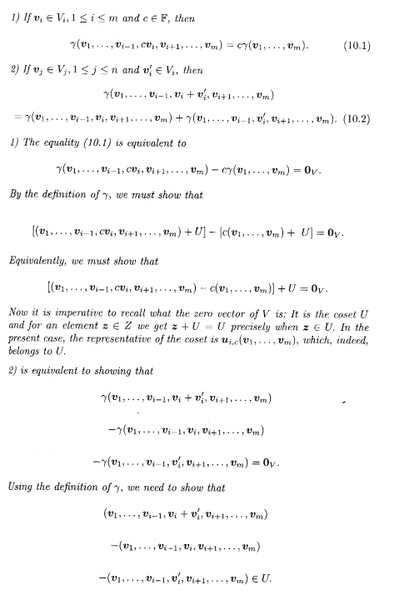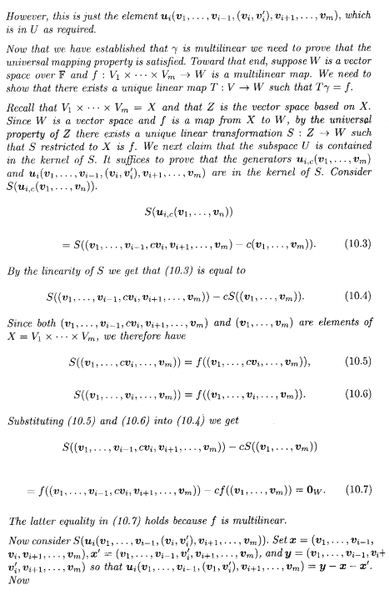In the above text we read the following:

" ... ... Recall that $V_1 \times \ ... \ \times V_m = X$ and that $Z$ is a vector space based on $X$. Since $W$ is a vector space and $f$ is a map from $X$ to $W$, by the universal property of $Z$ there exists a unique linear transformation $S \ : \ Z \longrightarrow W$ such that $S$ restricted to $X$ is $f$. ... ..."

Now I have summarised the mappings involved in Theorem 10.1 in Figure 1 below ... ...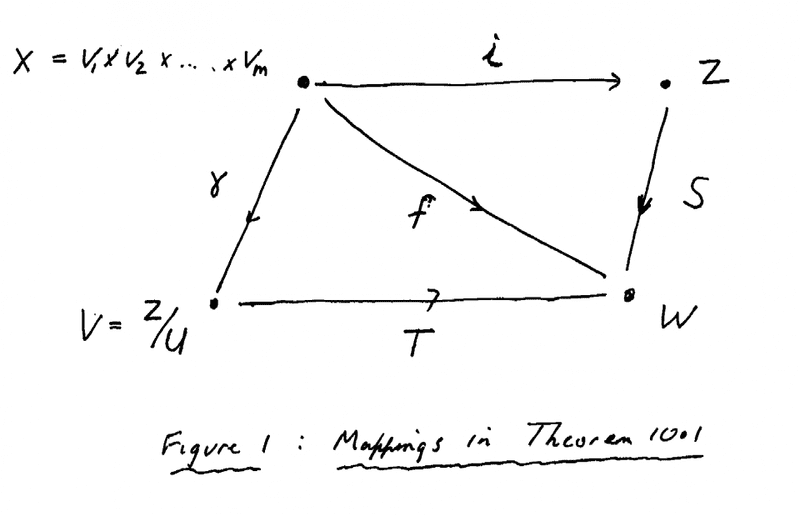My question is as follows:

Why does $Z$ have a universal mapping property ...? ... ... and indeed if $Z$ has one, why doesn't $V$ ... ... giving us the relationship $T \gamma = f$ that we want ... what is special about $Z$?

Hope someone can help ...

Peter

*** NOTE ***

... ... oh no! ... ... I think I have just realised the answer to my question ... hmm ... embarrassingly simple ... ... I think that $Z$ has a UMP because $( Z, \iota )$ is assumed to be the vector space based on the set $X$... and vector spaces based on a set have a UMP ... is that right? ... see Cooperstein Definition 10.1 on the first page of Section 10.1 provided below ...

Can someone confirm that this is the reason Z has a Universal Mapping Property ...

Peter

==========================================================

*** NOTE ***

It may help readers of the above post to be able to read Cooperstein's introduction to Section 10.1 where he covers, among other things, the notion of a vector space being based on a set and the idea of the universal mapping problem ... ... so I am providing this text as follows: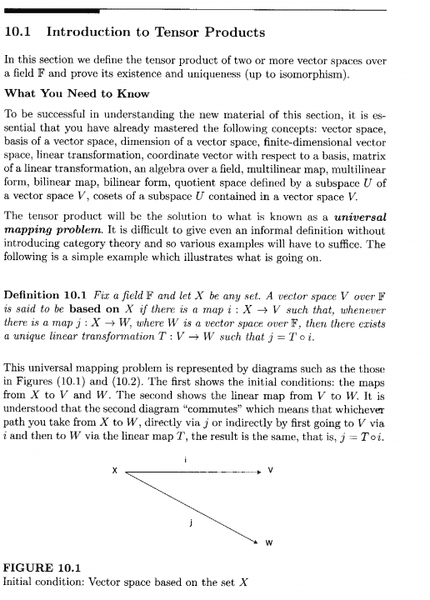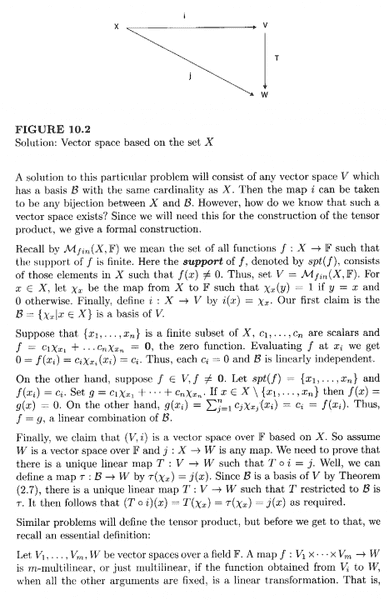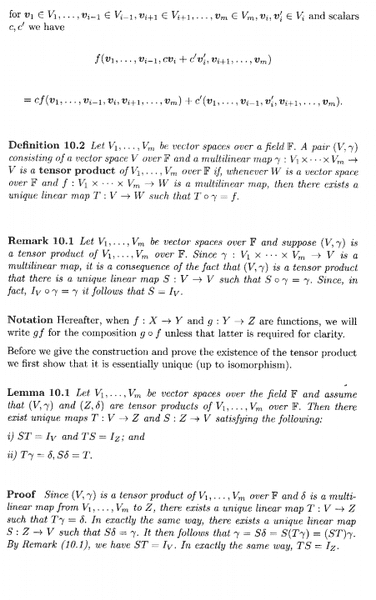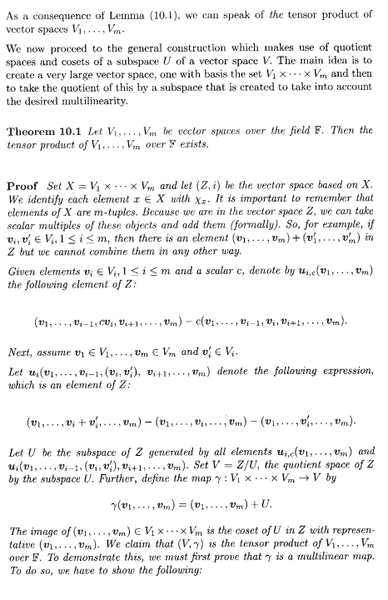Last edited:

## Answers and Replies

WWGD
Science Advisor
Gold Member
I think a helpful thing to know/understand is the concept of maps factoring through (the quotient, here): https://en.wikipedia.org/wiki/List_of_mathematical_jargon in algebra, there are conditions on the kernel of a map. Factoring through is in analogy with the factoring of numbers as products.

You are given functions f:A-->C , g: A-->B . Then f factors through g if there is an h: with f=hg ; h is a map from B-->C. This is I think the clearest way of understanding the tensor product. For vector spaces V,W , the tensor product , ## V \ Oline W ## is a vector space in which every bilinear map defined on VxW into a third vector space Z factors through a linear map from the tensor product into Z. The conditions on the kernel guarantee that maps factor through. I will look up the conditions on the kernel and image of the respective groups and get back with it.

•Math Amateur
Gold Member
Thanks WWGD ... most helpful ...

Peter

WWGD
Science Advisor
Gold Member
Thanks WWGD ... most helpful ...

Peter
Glad it helped, Peter, I went through my own pain trying to understand it -- I feel your pain :).

Gold Member
Thanks again WWGD ... good to have your support ...

Peter

•WWGD